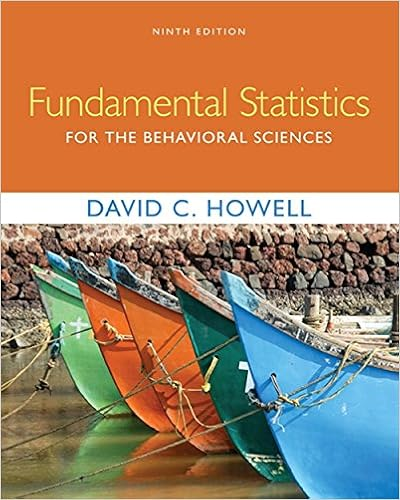# True or False: For all two-sample tests, the sample sizes...

• 20
• 86% (70) 60 out of 70 people found this document helpful

This preview shows page 1 - 3 out of 20 pages.

##### We have textbook solutions for you!
The document you are viewing contains questions related to this textbook.The document you are viewing contains questions related to this textbook.
Chapter 14 / Exercise 14.22
Fundamental Statistics for the Behavioral Sciences
HowellExpert Verified
Chapter 12 - Two-Sample Tests 1) True or False: For all two-sample tests, the sample sizes must be equal in the two groups.
2) True or False: When the sample sizes are equal, the pooled variance of the two groups is the average of the two sample variances.
3) The t test for the difference between the means of two independent populations assumes that the respective C
4) If we are testing for the difference between the means of two independent populations presuming equal variances with samples of n 1 = 20 and n 2 = 20, the number of degrees of freedom is equal to B
5) In testing for the differences between the means of two independent populations where the variances in each population are unknown but assumed equal, the degrees of freedom are A) n - 1. B) n 1 + n 2 - 1. C) n 1 + n 2 - 2. D) n - 2. Answer: C
6) In testing for differences between the means of two independent populations, the null hypothesis is: B
7) In testing for the differences between the means of two independent populations, you assume that the two populations each follow a ________ distribution.
8) Given the following information, calculate the degrees of freedom that should be used in the pooled- variance t test. 1
##### We have textbook solutions for you!
The document you are viewing contains questions related to this textbook.The document you are viewing contains questions related to this textbook.
Chapter 14 / Exercise 14.22
Fundamental Statistics for the Behavioral Sciences
HowellExpert Verified
S 1 2 = 4, S 2 2 = 6, n 1 = 16, n 2 = 25 B
9) Given the following information, calculate S p 2 , the pooled sample variance that should be used in the pooled-variance t test. S 1 2 = 4, S 2 2 = 6, n 1 = 16, n 2 = 25 A) S p 2 = 6.00 B) S p 2 = 5.00 C) S p 2 = 5.23 D) S p 2 = 4.00 Answer: C
10) True or False: A statistics professor wanted to test whether the grades on a statistics test were the same for upper and lower classmen. The professor took a random sample of size 10 from each, conducted a test, and found out that the variances were equal. For this situation, the professor should use a t test with related samples.
11) True or False: A statistics professor wanted to test whether the grades on a statistics test were the same for upper and lower classmen. The professor took a random sample of size 10 from each, conducted a test, and found out that the variances were equal. For this situation, the professor should use a t test with independent samples.
TABLE 1 Are Japanese managers more motivated than American managers? A randomly selected group of each were administered the Sarnoff Survey of Attitudes Toward Life (SSATL), which measures motivation for upward mobility. The SSATL scores are summarized below. American Japanese Sample Size 211 100 Sample Mean SSATL Score 65.75 79.83 Sample Std. Dev. 11.07 6.41 12) Referring to Table 1, judging from the way the data were collected, which test would likely be most appropriate to employ? B
2
•••